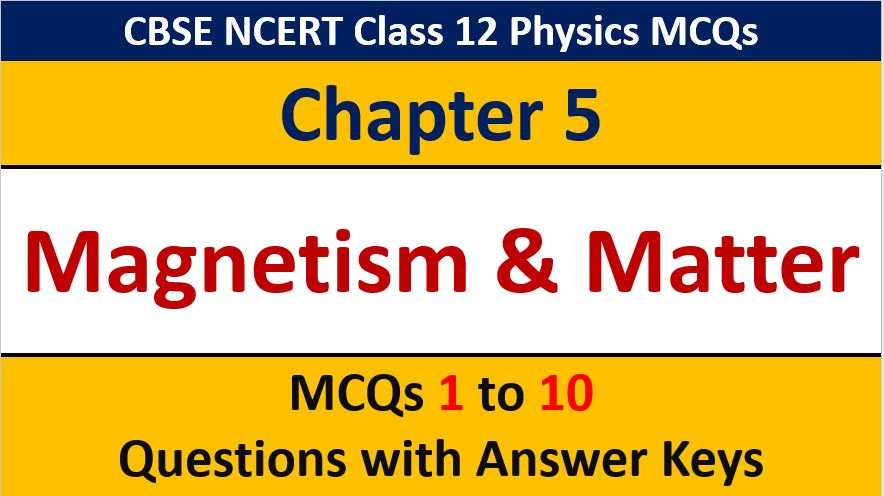## MCQ Questions for Class 12 Physics Chapter 5 Magnetism and Matter with Answer Keys

If you are preparing for CBSE Class 12 Board Exam Term 1 which will be an objective type questions paper, then you need to practice multiple choice questions of Class 12 Physics Chapterwise. In this article of AKVTutorials, you will get MCQ Questions for Class 12 Physics Chapter 5 Magnetism & Matter with Answer Keys.

MCQ Questions for Class 12 Physics Chapter 5 Question No 1:

B/ μ0 has the unit of

Option A : Energy

Option B : Energy density

Option C : Potential

Option D : Capacity

Option B : Energy density

MCQ Questions for Class 12 Physics Chapter 5 Question No 2:

Earth’s magnetic field always has a horizontal component except at

Option A : Equator

Option B : Magnetic pole

Option C : A latitude of 600

Option D : An inclination of 600

Option B : Magnetic pole

MCQ Questions for Class 12 Physics Chapter 5 Question No 3:

Magnetic meridian is a

( Ⅰ ) Point

( Ⅱ ) Line along E-W

( Ⅲ ) Vertical plane

( Ⅳ ) Horizontal plane

Option A : ( Ⅰ ) and ( Ⅲ )are correct

Option B :  ( Ⅰ ) and ( Ⅱ ) are correct

Option C : ( Ⅲ ) is correct

Option D : ( Ⅰ ), ( Ⅱ ) and ( Ⅳ ) are correct

Option C : ( Ⅲ ) is correct

MCQ Questions for Class 12 Physics Chapter 5 Question No 4:

The electric and magnetic field differ in that

Option A : Electric lines of force are closed curves while magnetic field lines are not.

Option B : Magnetic field lines are closed while electric lines are not

Option C : Electric line of force can give direction of the electric field while magnetic lines can not

Option D : Magnetic lines can give direction of magnetic field while electric lines can not

Option B : Magnetic field lines are closed while electric lines are not

MCQ Questions for Class 12 Physics Chapter 5 Question No 5:

When a bar magnet falls in a metal cylinder with vertical axis , the acceleration of the magnet is

Option A : = g

Option B : < g

Option C : > g

Option D : Zero

Option B : r< g

MCQ Questions for Class 12 Physics Chapter 5 Question No 6:

A steel wire of length L has a magnetic moment M. Then it is bent into a semi circular arc, then the new magnetic moment of the are

Option A : M

Option B : 2M / π

Option C : M / L

Option D : ML

Option B : 2M / π

MCQ Questions for Class 12 Physics Chapter 5 Question No 7:

When a bar magnet is heated, its magnetic moment

Option A : decreases

Option B : increases

Option C : remains same

Option D : none

Option A : decreases

MCQ Questions for Class 12 Physics Chapter 5 Question No 8:

Three identical bar magnets each of magnetic moment M are arranged in the form of an equilateral triangle such that unlike poles are contact. The resultant magnetic moment is

Option A :√ 3 M

Option B : M / 3

Option C : 3 M

Option D : Zero

Option D : Zero

MCQ Questions for Class 12 Physics Chapter 5 Question No 9:

A) When ‘n’ identical magnets are arranged in the form of closed polygon with unlike poles nearer, the resultant magnetic moment is Zero

B) If one magnet is removed from the above resultant magnetic moment becomes ‘M’

C) If one magnet is reversed in the polygon, the resultant magnetic moment of combination becomes 2M

Option A : A, B and C are correct

Option B : A and B are correct but C is wrong

Option C : Only A is correct

Option D : A, B and C are wrong

Option A : A, B and C are correct

MCQ Questions for Class 12 Physics Chapter 5 Question No 10:

The total number of magnetic lines of force origination or terminating on a pole of strength ‘m’ is

Option A : μ0m / 4π

Option B : m / μ0

Option C : m2

Option D : μ0m

Option D : μ0m

CBSE Class 12 Physics Chapterwise MCQ
1 : Electric Charge & Fields MCQs
| 1 to 10 | 11 to 20
2 : Electrostatics Potential and Capacitance MCQs
| 1 to 10 | 11 to 20
3 : Current Electricity MCQs
| 1 to 10 | 11 to 20
4 : Moving charges and Magnetism MCQs
| 1 to 10 | 11 to 20
5 : Magnetism and Matter MCQs
| 1 to 10 | 11 to 20
6 : Electromagnetic Induction MCQs
| 1 to 10 | 11 to 20
7 : Alternating Current MCQs
| 1 to 10 | 11 to 20
8 : Electromagnetic Waves MCQs
| 1 to 10 | 11 to 20
9 : Ray Optics and Optical Instruments MCQs
| 1 to 10 | 11 to 20
10 : Wave Optics MCQs
| 1 to 10 | 11 to 20
11 : Dual Nature of Radiation MCQs
| 1 to 10 | 11 to 20
12 : Atoms MCQs
| 1 to 10 | 11 to 20
13 : Nuclei MCQs
| 1 to 10 | 11 to 20
14 : Semi Conductor Electronics MCQs
| 1 to 10 | 11 to 20
15 : Communication Systems MCQs
| 1 to 10 | 11 to 20